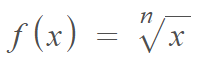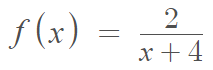# Implied Domain

In general, the implied domain of a function is the set of all real numbers for which the function is defined . If we’re given a function and the domain isn’t specified, we assume that the domain is the largest possible subset of real numbers: (-∞, ∞).

However, there are some notable exceptions .

1. The radicand of a square root function (the expression contained within the square root) must be zero or greater.
2. The denominator in a rational function cannot equal zero.
3. Logarithmic functions must have positive real numbers in the domain, because we cannot take the logarithm of negative numbers.has an implied domain of all reals if n is an even numbered natural number (i.e. the counting numbers 2, 4, 6,….). However, if n is an odd numbered natural number, we can’t take even roots of negative numbers so the implied domain is [0, ∞).

## Implied Domain: Examples

Example #1: (Radical Function) Find the implied domain of f(x) = √(x – 2).

Step 1: Set the radicand equal to or greater than zero:
(x – 2) ≥ 0.

Step 2: Solve for x:
x ≥ 2.

This tells us that the implied domain is the set of all real numbers greater than or equal to 2. In interval notation, we can write that as [2, ∞).

Example #2 (Rational Function): Find the implied domain of the following function:Step 1: Solve the denominator for x.

• x + 4 ≠ 0
• x ≠ -4.

This gives a domain of all real numbers except for -4. In interval notation, the domain is (-∞ -4) ∪ (-4, ∞).

Example #3: (Polynomial Function): What is the implied domain of f(x) = x2 + 5x?
Solution: All polynomial functions have an implied domain of all real numbers. We can write that in interval notation as (-∞, ∞). Or, we can say the domain is ℝ.

## A Practical Example

Sometimes you may be asked to find an implied domain for a word problem instead of an algebraic equation. For example, this precalculus worksheet asks you for the implied domain for a function concerning the monthly cost in dollars of a phone call. The input, “x” is the number of minutes used. As there are 43,200 minutes in a month, the longest possible phone call is 43,200 minutes and the shortest call is 0 minutes, giving an implied domain of [0, 43200].

## References

 Functions. Retrieved October 31, 2021 from: https://people.richland.edu/james/lecture/m116/functions/functions.html
 Handout. Retrieved October 31, 2021 from: https://somerset.kctcs.edu/current-students/media/student-support-services/workshops/implied-domain-handout.docx

CITE THIS AS:
Stephanie Glen. "Implied Domain" From StatisticsHowTo.com: Elementary Statistics for the rest of us! https://www.statisticshowto.com/implied-domain/